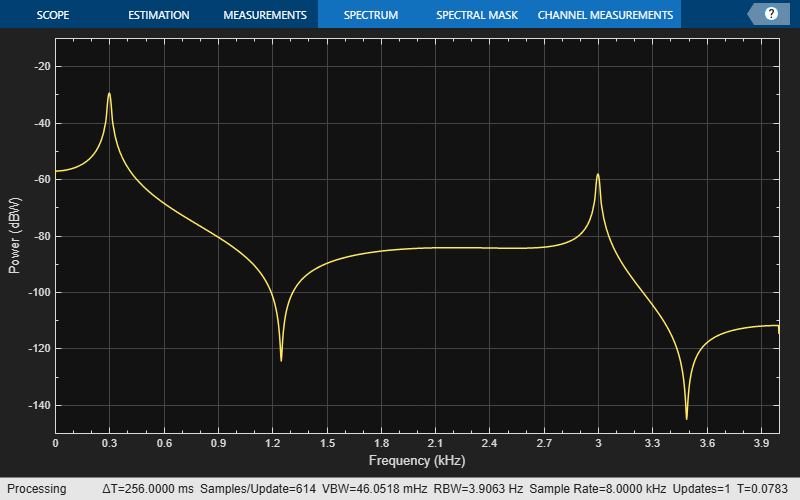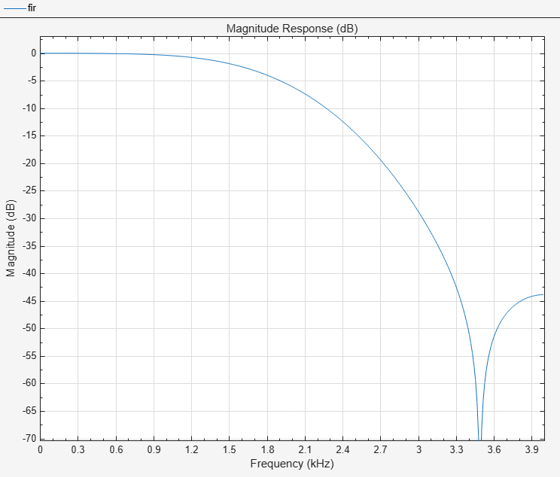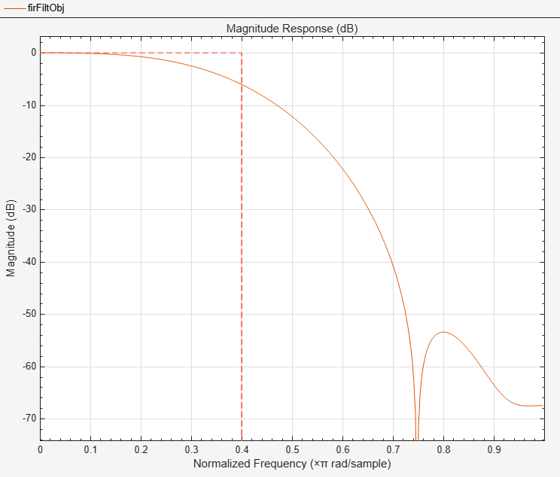Documentation

# dsp.FIRFilter

Static or time-varying FIR filter

## Description

The `dsp.FIRFilter` System object™ filters each channel of the input using static or time-varying FIR filter implementations.

To filter each channel of the input:

1. Create the `dsp.FIRFilter` object and set its properties.

2. Call the object with arguments, as if it were a function.

To learn more about how System objects work, see What Are System Objects? (MATLAB).

## Creation

### Syntax

``fir = dsp.FIRFilter``
``fir = dsp.FIRFilter(num)``
``fir = dsp.FIRFilter(Name,Value)``

### Description

````fir = dsp.FIRFilter` returns a finite impulse response (FIR) filter object, `fir`, which independently filters each channel of the input over time using a specified FIR filter implementation.```

example

````fir = dsp.FIRFilter(num)` returns an FIR filter System object, `fir`, with the `Numerator` property set to `num`.```
````fir = dsp.FIRFilter(Name,Value)` returns an FIR filter System object, `fir`, with each property set to the specified value.```

## Properties

expand all

Unless otherwise indicated, properties are nontunable, which means you cannot change their values after calling the object. Objects lock when you call them, and the `release` function unlocks them.

If a property is tunable, you can change its value at any time.

For more information on changing property values, see System Design in MATLAB Using System Objects (MATLAB).

Specify the filter structure. You can specify the filter structure as one of `Direct form` | `Direct form symmetric` | `Direct form antisymmetric` | ```Direct form transposed``` | `Lattice MA`.

Specify the source of the filter coefficients as `Property` or `Input port`. When you specify `Input port`, the filter object updates the time-varying filter once every frame.

#### Dependencies

This applies when you set the `Structure` to `Direct form` | `Direct form symmetric` | `Direct form antisymmetric` | ```Direct form transposed```.

Specify the source of the Lattice filter coefficients as `Property` or `Input port`. When you specify `Input port`, the filter object updates the time-varying filter once every frame.

#### Dependencies

This applies when you set the `Structure` to `Lattice MA`.

Specify the filter coefficients as a real or complex numeric row vector.

Tunable: Yes

#### Dependencies

This property applies when you set the `NumeratorSource` property to `Property`, and the Structure property is set to ```Direct form```, `Direct form symmetric`, ```Direct form antisymmetric```, or `Direct form transposed`.

Data Types: `single` | `double` | `int8` | `int16` | `int32` | `int64` | `uint8` | `uint16` | `uint32` | `uint64`
Complex Number Support: Yes

Specify the reflection coefficients of a lattice filter as a real or complex numeric row vector.

Tunable: Yes

#### Dependencies

This property applies when you set the Structure property to ```Lattice MA```, and the `ReflectionCoefficientsSource` property to `Property`.

Data Types: `single` | `double` | `int8` | `int16` | `int32` | `int64` | `uint8` | `uint16` | `uint32` | `uint64`
Complex Number Support: Yes

Specify the initial conditions of the filter states. The number of states or delay elements equals the number of reflection coefficients for the lattice structure, or the number of filter coefficients–1 for the other direct form structures.

You can specify the initial conditions as a scalar, vector, or matrix. If you specify a scalar value, the FIR filter object initializes all delay elements in the filter to that value. If you specify a vector whose length equals the number of delay elements in the filter, each vector element specifies a unique initial condition for the corresponding delay element. The object applies the same vector of initial conditions to each channel of the input signal.

If you specify a vector whose length equals the product of the number of input channels and the number of delay elements in the filter, each element specifies a unique initial condition for the corresponding delay element in the corresponding channel.

If you specify a matrix with the same number of rows as the number of delay elements in the filter, and one column for each channel of the input signal, each element specifies a unique initial condition for the corresponding delay element in the corresponding channel.

Tunable: Yes

Data Types: `single` | `double` | `int8` | `int16` | `int32` | `int64` | `uint8` | `uint16` | `uint32` | `uint64`

### Fixed-Point Properties

Specify whether to use full precision rules. If you set `FullPrecisionOverride` to `true`, which is the default, the object computes all internal arithmetic and output data types using full precision rules. These rules provide the most accurate fixed-point numerics. It also turns off the display of other fixed-point properties because they do not apply individually. These rules guarantee that no quantization occurs within the object. Bits are added, as needed, to ensure that no roundoff or overflow occurs. If you set `FullPrecisionOverride` to `false`, fixed-point data types are controlled through individual fixed-point property settings. For more information, see Full Precision for Fixed-Point System Objects.

Specify the rounding method.

#### Dependencies

This property applies only if the object is not in full precision mode.

Specify the overflow action as `Wrap` or `Saturate`.

#### Dependencies

This property applies only if the object is not in full precision mode.

Specify the coefficients fixed-point data type as ```Same word length as input``` or `Custom`.

#### Dependencies

This property applies when you set the `NumeratorSource` property to `Property`.

Specify the coefficients fixed-point type as a signed or unsigned `numerictype` object.

#### Dependencies

This property applies when you set the `CoefficientsDataType` property to `Custom`.

Specify the reflection coefficients fixed-point data type as ```Same word length as input``` or `Custom`.

#### Dependencies

This property applies when you set the `ReflectionCoefficientsSource` property to `Property`.

Specify the reflection coefficients fixed-point type as a signed or unsigned `numerictype` object.

#### Dependencies

This property applies when you set the `ReflectionCoefficientsDataType` property to `Custom`.

Specify the product fixed-point data type as `Full precision`, `Same as input`, or `Custom`.

Specify the product fixed-point type as a signed or unsigned scaled `numerictype` object.

#### Dependencies

This property applies when you set the `ProductDataType` property to `Custom`.

Specify the accumulator fixed-point data type to ```Full precision```, `Same as input`, ```Same as product```, or `Custom`.

Specify the accumulator fixed-point type as a signed or unsigned scaled `numerictype` object.

#### Dependencies

This property applies when you set the `AccumulatorDataType` property to `Custom`.

Specify the state fixed-point data type as one of ```Same as input```, `Same as accumulator`, or `Custom`.

#### Dependencies

This property does not apply to any of the direct form or direct form I filter structures.

Specify the state fixed-point type as a signed or unsigned scaled `numerictype` object.

#### Dependencies

This property applies when you set the `StateDataType` property to `Custom`.

Specify the output fixed-point data type as one of ```Same as input```, `Same as accumulator`, or `Custom`.

Specify the output fixed-point type as a signed or unsigned scaled `numerictype` object.

#### Dependencies

This property applies when you set the OutputDataType property to `Custom`.

## Usage

For versions earlier than R2016b, use the `step` function to run the System object algorithm. The arguments to `step` are the object you created, followed by the arguments shown in this section.

For example, `y = step(obj,x)` and `y = obj(x)` perform equivalent operations.

### Syntax

``y = fir(x)``
``y = fir(x,coeff)``

### Description

example

````y = fir(x)` applies an FIR filter to the real or complex input signal `x` to produce the output `y`.```
````y = fir(x,coeff)` uses the time-varying coefficients, `coeff` , to filter the input signal `x` and produce the output `y` . You can use this option when you set the `NumeratorSource` or `ReflectionCoefficientsSource` property to ```Input port```.```

### Input Arguments

expand all

Data input, specified as a vector or a matrix. When the input data is of a fixed-point type, it must be signed when the structure is set to ```Direct form symmetric``` or `Direct form antisymmetric`. The FIR filter object operates on each channel of the input signal independently over successive calls to step method.

This System object supports variable-size input.

Data Types: `single` | `double` | `int8` | `int16` | `int32` | `uint8` | `uint16` | `uint32` | `fi`
Complex Number Support: Yes

Time-varying filter coefficients, specified as a row vector. The data and coefficient inputs must have the same data type.

Data Types: `single` | `double` | `int8` | `int16` | `int32` | `uint8` | `uint16` | `uint32` | `fi`
Complex Number Support: Yes

### Output Arguments

expand all

Filtered output, returned as a vector or a matrix. The output has the same size and data type as the input.

Data Types: `single` | `double` | `int8` | `int16` | `int32` | `uint8` | `uint16` | `uint32` | `fi`
Complex Number Support: Yes

## Object Functions

To use an object function, specify the System object as the first input argument. For example, to release system resources of a System object named `obj`, use this syntax:

`release(obj)`

expand all

 `freqz` Frequency response of filter `fvtool` Visualize frequency response of DSP filters `impz` Impulse response of discrete-time filter System object `info` Information about filter System object `coeffs` Filter coefficients `cost` Estimate cost for implementing filter System objects `grpdelay` Group delay response of discrete-time filter System object `generatehdl` Generate HDL code for quantized DSP filter (requires Filter Design HDL Coder)
 `step` Run System object algorithm `release` Release resources and allow changes to System object property values and input characteristics `reset` Reset internal states of System object

## Examples

expand all

Use an FIR filter to apply a low pass filter to a waveform with two sinusoidal components.

``` t = (0:1000)'/8e3; xin = sin(2*pi*0.3e3*t)+sin(2*pi*3e3*t); sr = dsp.SignalSource; sr.Signal = xin; sink = dsp.SignalSink; fir = dsp.FIRFilter(fir1(10,0.5)); sa = dsp.SpectrumAnalyzer('SampleRate',8e3,... 'PlotAsTwoSidedSpectrum',false,... 'OverlapPercent', 80, 'PowerUnits','dBW',... 'YLimits', [-150 -10]); while ~isDone(sr) input = sr(); filteredOutput = fir(input); sink(filteredOutput); sa(filteredOutput) end filteredResult = sink.Buffer; fvtool(fir,'Fs',8000) ```Design an FIR filter as a System object.

```N = 10; Fc = 0.4; B = fir1(N,Fc); fir1 = dsp.FIRFilter(B); fvtool(fir1) ```This can also be achieved by using `fdesign` as a constructor and `design` to design the filter.

```N = 10; Fc = 0.4; specLowpass = fdesign.lowpass('N,Fc',N,Fc); fir2 = design(specLowpass,'systemobject',true) fvtool(fir2); ```
```fir2 = dsp.FIRFilter with properties: Structure: 'Direct form' NumeratorSource: 'Property' Numerator: [1x11 double] InitialConditions: 0 Use get to show all properties ```## Algorithms

This object implements the algorithm, inputs, and outputs described on the Discrete FIR Filter block reference page. The object properties correspond to the block parameters.

Watch now